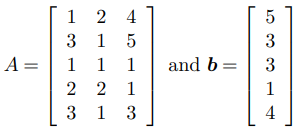# Find the least squares solution of the inconsistent linear system

## Description

Find the least-squares solution of the inconsistent linear system Ax = b, where:The normal equations are given bywhere,The least squares solution xˆ is obtained by solving the normal equations. In MATLAB:

>> A = [1 2 4; 3 1 5; 1 1 1; 2 2 1; 3 1 3]

>> b = [5;3;3;1;4]

>> xh = A’*A\(A’*b)
xh =
-0.1639
0.8970
0.7507

In Python, the solution of the least-squares problem is obtained by using the following Python commands:

import numpy as np

A = np.array([[1, 2, 4], [3, 1, 5], [1, 1, 1],[2, 2, 1], [3, 1, 3]])

b = np.array([,,,,])

xh = np.linalg.solve([email protected], [email protected])

print(xh)

## Reviews

There are no reviews yet.

Categories: ,